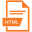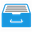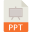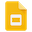AMSC460 / CMSC460 Computational Methods Spring 2015## Course Information

 Instructor Changhui Tan Lectures Tu Th 2:00pm - 3:15pm, at MATH0201 Office CSIC 4123 Office Hours Tu Th 11:00am - 12:00pm, or by appointment Textbooks (Required) E. Suli and D. Mayers, An introduction to numerical analysis. (ISBN: 9780521007948) C. Moler, Numerical computing with Matlab. (ISBN: 9780898716603)   [Online copy]

## Course Description

• The official Math Department course description on AMSC/CMSC 460.
 Topic References » Linear systems of equations [Suli] Chapter 2, [Moler] Chapter 2. Note #1 on Gauss elimination[HTML][PDF]. Last update: 2/2, typo spotted by Frank VanGessel. » Least square problems [Suli] Chapter 5, [Moler] Chapter 5. » Root finder [Suli] Chapter 1,4, [Moler] Chapter 4. » Polynomial approximation [Suli] Chapter 6,9,11, [Moler] Chapter 3. Note #2 on Polynomial interpolation[HTML][PDF]. Last update: 3/23. » Numerical integration [Suli] Chapter 7,10, [Moler] Chapter 6. » Numerical ODE [Suli] Chapter 12, [Moler] Chapter 7. » Finite element method [Suli] Chapter 14.

## Homework Assignments

• 10 homework assignments will be given during the semester. The lowest score will be dropped.
• Homework 9 available. It is due on 4/30 in class. Last update: 4/21.
•  Homework # 1 2 3 4 5 6-7 8 9 10 Due 2/10 2/19 3/3 3/12 3/26 4/9 4/21 4/30 --
•Download Homework Assignments.
• Additional resources for the homework.
• » Homework 9: a Matlab script on multistep methods: multistep.m. Last update: 4/21.
• » Homework 6-7: a Matlab script for linear spline least square approximation: linearspline.m. Last update: 4/1.
• » Homework 5: a Matlab code to test if your code on Thomas algorithm is correct: testthomas.m. Last update: 3/12.
• » Homework 4: a Matlab code on Runge's phenomenon: runge.m. Last update: 3/3.
• » Homework 1: a Matlab code on Gauss elimination (without pivoting): mylu.m. Last update: 1/29.
•Download Additional Resources.

## Group Project

• Groups has been assigned. Please go to canvas to see your assigned project and groupmates. Last update: 3/12.
• There are five group projects. The brief discription can be found HERE. The project is due at the end of the semester, including an in-class presentation and a report.
•  Group # Project title Presentation Report 1 Iterative methods for linear sparse system[Slides - PPT][PDF] 2 Eigen-systems for large matrices[Slides - Google][PDF] 3 Polynomial approximation: minimizing infinity-norm[Slides - PPT][PDF] 4 Extrapolation in numerical integration[Slides - PDF][PDF] 5 Numerical methods for boundary value problems of second order ODE[Slides - PPT][PDF]

## Exams

• There are three midterm exams during the semester. A final exam will be given at the end of the semester.
• The final exam will be held on May 18 (Monday) from 10:30 to 12:30 in MATH0201.
•  .Exam #. .Schedule. .Contents. .Results. 1 2/26 Linear system, Least square, Root finder Max 102, Min 40, Median 75. 90+ 7, 80+ 6, 70+ 8, 60+ 8, 60- 3. 2 Due 4/14 Polynomial approximation, Numerical integration Max 100, Min 34, Median 87. 100 3, 90+ 9, 80+ 7, 70+ 6, 60+ 3, 60- 1. 3 4/30 Numerical ODE, Finite element method Max 97, Min 25, Median 71. (Will curve up by 7) 90+ 3, 80+ 6, 70+ 7, 60+ 5, 60- 8. Final 5/18 Cumulative
•Download Exams and Solutions.